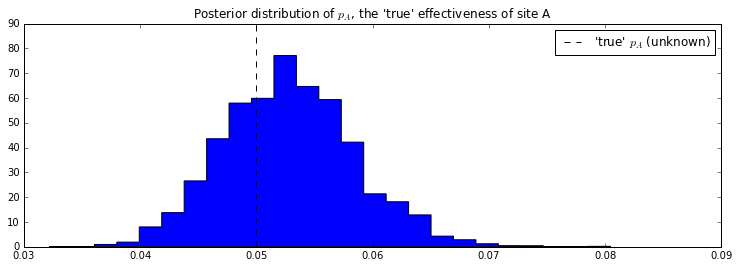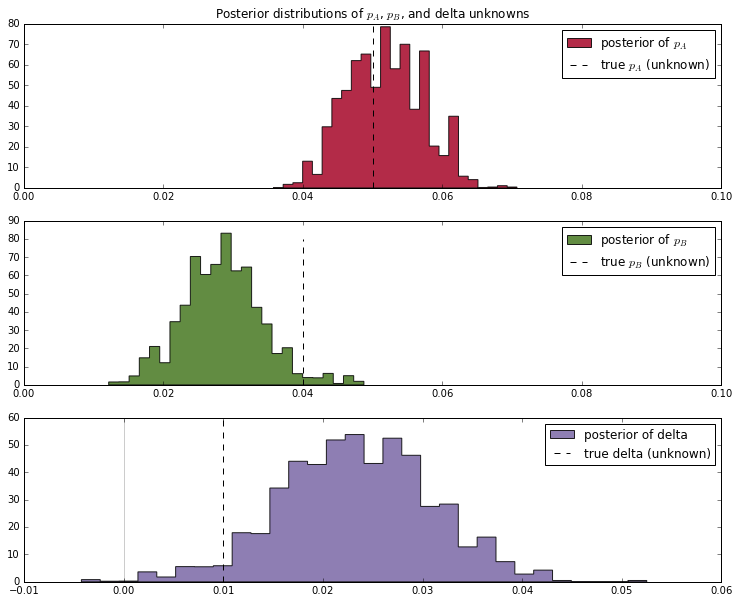AB-Testing slides

### AB Testing¶

In :
# set constants
p_true = 0.05  # remember, this is unknown.
N = 1500

# sample N Bernoulli random variables from Ber(0.05).
# each random variable has a 0.05 chance of being a 1.
# this is the data-generation step
occurrences = pm.rbernoulli(p_true, N)

print occurrences  # Remember: Python treats True == 1, and False == 0
print occurrences.sum()

[False False False ..., False False False]
78


#### Maximum Likelihood Approach¶

In :
# Occurrences.mean is equal to n/N.
print "What is the observed frequency in Group A? %.4f" % occurrences.mean()
print "Does this equal the true frequency? %s" % (occurrences.mean() == p_true)

What is the observed frequency in Group A? 0.0520
Does this equal the true frequency? False

In :
occurrences

Out:
array([False, False, False, ..., False, False, False], dtype=bool)
In :
# Model for A:
# The parameters are the bounds of the Uniform.
p = pm.Uniform('p', lower=0, upper=1)
# include the observations, which are Bernoulli
obs = pm.Bernoulli("obs", p, value=occurrences, observed=True)

# To be explained later
mcmc = pm.MCMC([p, obs])
mcmc.sample(18000, 1000)

 [-----------------100%-----------------] 18000 of 18000 complete in 1.8 sec
In :
figsize(12.5, 4)
plt.title("Posterior distribution of $p_A$, the 'true' effectiveness of site A")
plt.vlines(p_true, 0, 90, linestyle="--", label="'true' $p_A$ (unknown)")
plt.hist(mcmc.trace("p")[:], bins=25, histtype="stepfilled", normed=True)
plt.legend()

Out:
<matplotlib.legend.Legend at 0x111931250>#### A vs. B¶

In :
import pymc as pm
figsize(12, 4)

# these two quantities are unknown to us.
true_p_A = 0.05
true_p_B = 0.04

# notice the unequal sample sizes -- no problem in Bayesian analysis.
N_A = 1500
N_B = 750

# generate some observations
observations_A = pm.rbernoulli(true_p_A, N_A)
observations_B = pm.rbernoulli(true_p_B, N_B)
print "Obs from Site A: ", observations_A[:30].astype(int), "..."
print "Obs from Site B: ", observations_B[:30].astype(int), "..."

Obs from Site A:  [0 0 0 0 0 0 0 0 0 0 0 0 0 0 0 0 0 0 0 0 0 0 0 0 0 0 0 0 0 0] ...
Obs from Site B:  [0 0 0 1 0 0 0 0 0 0 0 0 0 0 0 0 0 0 0 0 0 0 0 0 1 0 0 0 0 0] ...

In :
print observations_A.sum()
print observations_B.sum()

76
20

In :
print observations_A.mean()
print observations_B.mean()

0.0506666666667
0.0266666666667

In :
observations_A

Out:
array([False, False, False, ..., False, False, False], dtype=bool)
In :
def get_model(observations_A, observations_B):
# Set up the pymc model. Again assume Uniform priors for p_A and p_B.
p_A = pm.Uniform("p_A", 0, 1)
p_B = pm.Uniform("p_B", 0, 1)

# Define the deterministic delta function. This is our unknown of interest.
@pm.deterministic
def delta(p_A=p_A, p_B=p_B):
return p_A - p_B

# Set of observations, in this case we have two observation datasets.
obs_A = pm.Bernoulli("obs_A", p_A, value=observations_A, observed=True)
obs_B = pm.Bernoulli("obs_B", p_B, value=observations_B, observed=True)

return pm.Model([p_A, p_B, delta, obs_A, obs_B])

In :
model = get_model(observations_A, observations_B)
# To be explained in chapter 3.
mcmc = pm.MCMC(model)
mcmc.sample(10000, 100)

 [-----------------100%-----------------] 10000 of 10000 complete in 2.1 sec
In :
p_A_samples = mcmc.trace("p_A")[:]
p_B_samples = mcmc.trace("p_B")[:]
delta_samples = mcmc.trace("delta")[:]

In :
for i in range(10):
print p_A_samples[i], p_B_samples[i], delta_samples[i]

0.061542067665 0.0376602065622 0.0238818611028
0.061542067665 0.0376602065622 0.0238818611028
0.061542067665 0.0376602065622 0.0238818611028
0.061542067665 0.0376602065622 0.0238818611028
0.061542067665 0.0376602065622 0.0238818611028
0.061542067665 0.0376602065622 0.0238818611028
0.061542067665 0.0376602065622 0.0238818611028
0.061542067665 0.0376602065622 0.0238818611028
0.061542067665 0.0376602065622 0.0238818611028
0.061542067665 0.0376602065622 0.0238818611028

In :
figsize(12.5, 10)

# histogram of posteriors

ax = plt.subplot(311)

plt.xlim(0, .1)
plt.hist(p_A_samples, histtype='stepfilled', bins=25, alpha=0.85,
label="posterior of $p_A$", color="#A60628", normed=True)
plt.vlines(true_p_A, 0, 80, linestyle="--", label="true $p_A$ (unknown)")
plt.legend(loc="upper right")
plt.title("Posterior distributions of $p_A$, $p_B$, and delta unknowns")

ax = plt.subplot(312)

plt.xlim(0, .1)
plt.hist(p_B_samples, histtype='stepfilled', bins=25, alpha=0.85,
label="posterior of $p_B$", color="#467821", normed=True)
plt.vlines(true_p_B, 0, 80, linestyle="--", label="true $p_B$ (unknown)")
plt.legend(loc="upper right")

ax = plt.subplot(313)
plt.hist(delta_samples, histtype='stepfilled', bins=30, alpha=0.85,
label="posterior of delta", color="#7A68A6", normed=True)
plt.vlines(true_p_A - true_p_B, 0, 60, linestyle="--",
label="true delta (unknown)")
plt.vlines(0, 0, 60, color="black", alpha=0.2)
plt.legend(loc="upper right");In :
# Count the number of samples less than 0, i.e. the area under the curve
# before 0, represent the probability that site A is worse than site B.
print "Probability site A is WORSE than site B: %.3f" % \
(delta_samples < 0).mean()

print "Probability site A is BETTER than site B: %.3f" % \
(delta_samples > 0).mean()

Probability site A is WORSE than site B: 0.002
Probability site A is BETTER than site B: 0.998


### Same model in other form¶

Instead of using a Bernoulli variable with many observation for each webside, we could use a Binomial variable with the sufficent statistics $k_i$ and $n_i$ for describing the observed data.

In :
def get_model(observations_A, observations_B):

n_A = len(observations_A)
k_A = observations_A.sum()

n_B = len(observations_B)
k_B = observations_B.sum()

# Set up the pymc model. Again assume Uniform priors for p_A and p_B.
p_A = pm.Uniform("p_A", 0, 1)
p_B = pm.Uniform("p_B", 0, 1)

# Define the deterministic delta function. This is our unknown of interest.
@pm.deterministic
def delta(p_A=p_A, p_B=p_B):
return p_A - p_B

# Set of observations, in this case we have two observation datasets.
obs_A = pm.Binomial("obs_A", p=p_A, n = n_A, value=k_A, observed=True)
obs_B = pm.Binomial("obs_B", p=p_B, n = n_B, value=k_B, observed=True)

return pm.Model([p_A, p_B, delta, obs_A, obs_B])


#### Multiple Website designs¶

Problem: If we compare many designs with our current design, by change we get probably with our simple AB-Test a significant "better" design due to statistical fluctuations.

Let's simulate this (with the same true_p conversion rate for all designs).

In :
true_p = 0.05
N = 1000
# data of old website
observation_A = pm.rbernoulli(true_p, N)

# data of new website
num_designs = 8
observation_Bs = pm.rbernoulli(true_p, (num_designs, N))

In :
def many_designs(observation_A, observation_Bs):
diffs = np.ndarray(len(observation_Bs))
for i, observation_B in enumerate(observation_Bs):
model = get_model(observations_A, observation_B)
mcmc = pm.MCMC(model)
mcmc.sample(100000, 10000)
delta_samples = mcmc.trace("delta")[:]
print "\nProbability new design %d is BETTER than site A: %.3f" % \
(i, (delta_samples < 0.).mean())
diffs[i] = ((delta_samples < 0).mean())
return diffs

In :
print "MLE of p of site A", observation_A.mean()
print "MLE of p of site B", observation_Bs.mean(axis=1)
print "diff MLE of site B better than site A:", observation_Bs.mean(axis=1) - observation_A.mean()

MLE of p of site A 0.045
MLE of p of site B [ 0.056  0.052  0.047  0.048  0.054  0.052  0.055  0.043]
diff MLE of site B better than site A: [ 0.011  0.007  0.002  0.003  0.009  0.007  0.01  -0.002]

In :
diffs = many_designs(observation_A, observation_Bs)

 [-----------------100%-----------------] 100000 of 100000 complete in 11.0 sec
Probability new design 0 is BETTER than site A: 0.730
[-----------------100%-----------------] 100000 of 100000 complete in 8.1 sec
Probability new design 1 is BETTER than site A: 0.564
[-----------------100%-----------------] 100000 of 100000 complete in 9.4 sec
Probability new design 2 is BETTER than site A: 0.347
[-----------------100%-----------------] 100000 of 100000 complete in 8.5 sec
Probability new design 3 is BETTER than site A: 0.389
[-----------------100%-----------------] 100000 of 100000 complete in 7.7 sec
Probability new design 4 is BETTER than site A: 0.647
[-----------------100%-----------------] 100000 of 100000 complete in 7.8 sec
Probability new design 5 is BETTER than site A: 0.568
[-----------------100%-----------------] 100000 of 100000 complete in 7.8 sec
Probability new design 6 is BETTER than site A: 0.695
[-----------------100%-----------------] 100000 of 100000 complete in 8.0 sec
Probability new design 7 is BETTER than site A: 0.195

In :
diffs

Out:
array([ 0.72982222,  0.56365556,  0.34713333,  0.389     ,  0.6472    ,
0.56763333,  0.69466667,  0.19466667])

### Hierarchical Model¶

Idea: Sharing statistical strength between the individual versions (e.g. different webside designs).

#### General Hierachical Model¶

The posterior of the hidden variables given the data $Y$ is proportial to the likelihood times the prior (nominator in Bayes rule):

$$P(\theta, \phi \mid Y) \propto P(Y \mid \theta, \phi) P(\theta, \phi) = P(Y \mid \theta, \phi) P(\theta \mid \phi)P(\phi)$$

The data $Y$ is conditional independent of $\phi$ given $\theta$ so this becomes:

$$P(\theta, \phi \mid Y) \propto P(Y \mid \phi) P(\theta \mid \phi) P(\phi)$$

Note: The prior for our data $P(\theta \mid \phi)$ depends on another hidden variable $\phi$. That's called an hierarchical prior.

#### Hierachical Model for our website data¶

The data for each webside design $i$ can be described by the sufficent statistics ($k_i, n_i$).

Our model:

$$k_i \sim \textrm{Binomial}(\theta_i, n_i)$$$$\theta_i \sim \textrm{Beta}(\alpha, \beta)$$$$(\alpha, \beta) \sim p(\alpha, \beta)$$

Hierarchical Prior:

• $\phi = \{\alpha, \beta\}$

The $\alpha, \beta$ describes the variability of the different webside designs.

Which prior for $(\alpha, \beta)$ is appropriate? For a discussion see [Gel]

In :
pymc = pm

In :
def get_hierachical_model(observations):

N_s = np.array([len(i) for i in observations])
k_s = np.array([i.sum() for i in observations])
print "mle for p of each website:", [float(k)/n for k, n in zip(k_s, N_s)]

@pymc.stochastic(dtype=np.float64)
def beta_priors(value=[1.0, 1.0]):
a, b = value
if a <= 0 or b <= 0:
return -np.inf
else:
return np.log(np.power((a + b), -2.5))

a = beta_priors
b = beta_priors

# The hidden rate theta for each website.
thetas = pymc.Beta('thetas', a, b, size=len(observations))
observed_values = pymc.Binomial('observed_values', n=N_s, p=thetas, observed=True, value=k_s)

model = pymc.Model([a, b, thetas, observed_values])
return model

In :
def many_designs_with_hierachical_model(observation_A, observation_Bs):
diffs = np.ndarray(len(observation_Bs))
observations = [observation_A] + [ob for ob in observation_Bs]
model = get_hierachical_model(observations)
mcmc = pymc.MCMC(model)

# Generate 1,000,000 samples, and throw out the first 500,000
mcmc.sample(1000000, 500000)
for i in range(len(observation_Bs)):
diff = mcmc.trace('thetas')[:][:,0] - mcmc.trace('thetas')[:][:,i+1]

# in diffs prob of new site better than old one:
diffs[i] = (diff < 0).mean()
return diffs

In :
diffs_hierarchical = many_designs_with_hierachical_model(observation_A, observation_Bs)

mle for p of each website: [0.045, 0.056, 0.052, 0.047, 0.048, 0.054, 0.052, 0.055, 0.043]
[-----------------100%-----------------] 1000000 of 1000000 complete in 117.9 sec
In :
diffs_hierarchical

Out:
array([ 0.709996,  0.63362 ,  0.481302,  0.546752,  0.692246,  0.63767 ,
0.696962,  0.442184])
In :
diffs

Out:
array([ 0.72982222,  0.56365556,  0.34713333,  0.389     ,  0.6472    ,
0.56763333,  0.69466667,  0.19466667])